This is an archive of the old MediaWiki-based ImageJ wiki. The current website can be found at imagej.net.

Rand error

The Rand index is a well-known measure of the similarity between two data clusterings. Recently, it has been proposed as a measure of segmentation performance, since a segmentation can be regarded as a clustering of pixels. More formally, define a segmentation as an integer-valued labeling of an image. Each object in a segmentation consists of a set of pixels sharing a common label.

The Rand index is defined as a measure of agreement:

Given two segmentations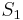$S_1$ and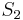$S_2$ of an image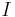$I$ with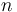$n$ pixels, we define:

•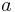$a$, the number of pairs of pixels in$I$ that are in the same object in$S_1$ and in the same object in$S_2$ (i.e., they have the same label)
•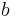$b$, the number of pairs of pixels in$I$ that are in different objects in$S_1$ and in different objects in$S_2$ (i.e., they have different labels)

The Rand index,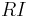$RI$, is: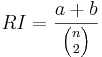$RI = \frac{a+b}{{n \choose 2 }}$

Here we instead define the closely related Rand error, which is a measure of disagreement. The Rand error (RE) is the frequency with which the two segmentations disagree over whether a pair of pixels belongs to same or different objects: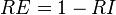$RE = 1 - RI$

Implementation in Fiji

The Rand error metric is implemented in the Trainable Weka Segmentation library. Here is an example of how to use it in a Beanshell script:

import trainableSegmentation.metrics.RandError;
import ij.IJ;

// original labels
originalLabels = IJ.openImage("/path/original-labels.tif");

// proposed (new) labels
proposedLabels = IJ.openImage("/path/proposed-labels.tif");

// threshold to binarize labels
threshold = 0.5;

metric = new RandError( originalLabels, proposedLabels );
randError = metric.getMetricValue( threshold );

IJ.log("Rand error between source image " + originalLabels.getTitle() + " and target image "
+ proposedLabels.getTitle() + " = " + randError);